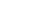News
Your location:Home > News > Industry dynamics

# Different frequency resonance in power system of series resonance

## Add time：2022-02-10 12:03:15   Number of views：76

Series resonances of different frequencies often occur in power systems, and their occurrences are actually over the inductance value Xm of the integrated inductance Xm of the capacitance Xco of the capacitance distributed between the wires and the ground in the power system and the parallel operation of the voltage transformer. The following is the performance of several different frequencies of resonance.

1. When the ratio of Xco is small, the resonance that occurs is frequency division resonance. Because in this case, the capacitance is relatively large, the energy exchange time when the capacitance and inductance oscillate is longer. If the number of energy exchanges within 1s is a fraction of the power frequency, such as 1/2, 1 /3, 1/4, etc., the resonance of this frequency becomes the frequency division resonance.

which performed:

1) The overvoltage multiple is low, generally not more than 2.5 times the phase voltage.

2) The indications of the three-phase voltmeter increase at the same time, and there are periodic swings; the indications of the line voltmeter basically remain unchanged.

2. When the ratio of Xco/Xm is large, the resonance that occurs is high frequency resonance. Because the capacitance to ground is relatively small at this time, the energy exchange time when the capacitor and inductance oscillate is short. If the number of energy exchanges within 1s is an integer multiple of the power supply frequency, such as 3, 5, 7 times of 50Hz, etc. , The resonance of this frequency becomes high frequency resonance.

which performed:

1) The overvoltage multiple is higher.

2) The indications of the three-phase voltmeter increase at the same time, and are much higher than the frequency division resonance, and the line voltage indications are the same as the frequency division resonance.

3) The overcurrent is small at resonance.

3. The ratio of Xco/Xm is between frequency division and high frequency, when it is close to 50Hz, the resonance that occurs is fundamental frequency resonance. When fundamental frequency resonance occurs, the number of energy exchanges of inductance and capacitance within 1S is exactly equal to the power frequency, so it becomes fundamental frequency resonance.

which performed:

1) In the three-phase voltmeter, the two-phase indicator increases and the one-phase decreases, and the line voltage is basically unchanged.

2) At resonance, the overcurrent is very large and the voltage transformer makes noise.

3) Generally, the overvoltage multiple does not exceed 3.2 times the phase voltage.

4) The fundamental frequency resonance is similar to the phenomenon when the system is single-phase grounded (false grounding phenomenon)

5) Often lead to equipment insulation breakdown, lightning arrester damage, transformer fuse blown, etc.

• Add：Power Valley Technology Center, 3088 Lekai North Street, Baoding City
• Phone：0086-15231260588 / 0086-13315299977
• Tel：0086-312-3172660 / 3188565
• E-mail：bdweisheng@126.com
•Mobile browsing

•Wechat attention